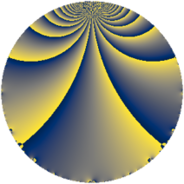# Properties

 Label 49.2.eLevel $49$ Weight $2$ Character orbit 49.e Rep. character $\chi_{49}(8,\cdot)$ Character field $\Q(\zeta_{7})$ Dimension $18$ Newform subspaces $2$ Sturm bound $9$ Trace bound $1$

# Related objects

## Defining parameters

 Level: $$N$$ $$=$$ $$49 = 7^{2}$$ Weight: $$k$$ $$=$$ $$2$$ Character orbit: $$[\chi]$$ $$=$$ 49.e (of order $$7$$ and degree $$6$$) Character conductor: $$\operatorname{cond}(\chi)$$ $$=$$ $$49$$ Character field: $$\Q(\zeta_{7})$$ Newform subspaces: $$2$$ Sturm bound: $$9$$ Trace bound: $$1$$ Distinguishing $$T_p$$: $$2$$

## Dimensions

The following table gives the dimensions of various subspaces of $$M_{2}(49, [\chi])$$.

Total New Old
Modular forms 30 30 0
Cusp forms 18 18 0
Eisenstein series 12 12 0

## Trace form

 $$18q - 5q^{2} - 3q^{3} - 5q^{4} - q^{5} - 9q^{6} + 5q^{8} - 14q^{9} + O(q^{10})$$ $$18q - 5q^{2} - 3q^{3} - 5q^{4} - q^{5} - 9q^{6} + 5q^{8} - 14q^{9} + 11q^{10} - 6q^{11} - 14q^{12} + 7q^{13} - 21q^{14} - 3q^{15} + 13q^{16} + 4q^{17} + 16q^{18} - 22q^{19} + 7q^{20} + 21q^{21} + 5q^{22} - 12q^{23} + 11q^{24} + 14q^{25} + 21q^{26} + 12q^{27} - 27q^{29} + 40q^{30} - 24q^{31} - 3q^{32} + 41q^{33} - 37q^{34} + 21q^{35} + q^{36} - 26q^{37} + 25q^{38} - 7q^{39} - 15q^{40} + 21q^{41} - 49q^{42} + 29q^{43} - 13q^{44} - 55q^{45} + 3q^{46} - 36q^{47} + 12q^{48} + 14q^{49} - 76q^{50} + 19q^{51} - 7q^{52} - 20q^{53} + 50q^{54} - 47q^{55} - 14q^{56} + 11q^{57} - 31q^{58} + 25q^{59} - 35q^{60} - 15q^{61} + 61q^{62} + 7q^{63} + 27q^{64} + 49q^{65} - 31q^{66} + 36q^{67} + 28q^{68} + 5q^{69} + 35q^{70} - 34q^{71} + 4q^{72} + 46q^{73} + 59q^{74} - 9q^{75} + 56q^{77} - 7q^{78} + 44q^{79} + 32q^{80} - 48q^{81} + 14q^{82} - 21q^{83} + 28q^{84} + 11q^{85} - 3q^{86} + 43q^{87} + 5q^{88} - q^{89} - 4q^{90} - 91q^{91} + 44q^{92} - 65q^{93} - 80q^{94} - 8q^{95} - 98q^{96} - 28q^{97} - 7q^{98} - 20q^{99} + O(q^{100})$$

## Decomposition of $$S_{2}^{\mathrm{new}}(49, [\chi])$$ into newform subspaces

Label Dim. $$A$$ Field CM Traces $q$-expansion
$$a_2$$ $$a_3$$ $$a_5$$ $$a_7$$
49.2.e.a $$6$$ $$0.391$$ $$\Q(\zeta_{14})$$ None $$-3$$ $$-3$$ $$6$$ $$7$$ $$q+(-1+\zeta_{14}-\zeta_{14}^{4}+\zeta_{14}^{5})q^{2}+\cdots$$
49.2.e.b $$12$$ $$0.391$$ $$\Q(\zeta_{21})$$ None $$-2$$ $$0$$ $$-7$$ $$-7$$ $$q+(\zeta_{21}^{2}+\zeta_{21}^{4}-\zeta_{21}^{5}-\zeta_{21}^{9}+\zeta_{21}^{11})q^{2}+\cdots$$